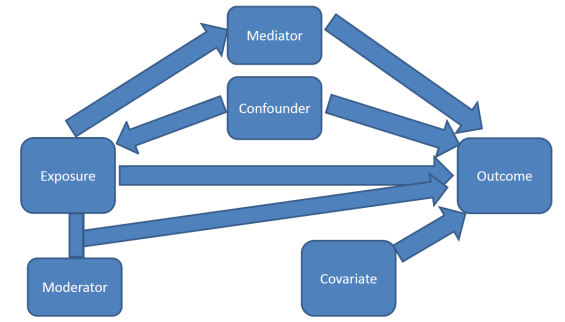## 9.6 Confounding

One primary purpose of a multivariable model is to assess the relationship between a particular explanatory variable $$x$$ and your response variable $$y$$, after controlling for other factors.All the ways covariates can affect response variables
Easy to read short article from a Gastroenterology journal on how to control confounding effects by statistical analysis. https://www.ncbi.nlm.nih.gov/pmc/articles/PMC4017459/

Other factors (characteristics/variables) could also be explaining part of the variability seen in $$y$$.

If the relationship between $$x_{1}$$ and $$y$$ is bivariately significant, but then no longer significant once $$x_{2}$$ has been added to the model, then $$x_{2}$$ is said to explain, or confound, the relationship between $$x_{1}$$ and $$y$$.

Steps to determine if a variable $$x_{2}$$ is a confounder.

1. Fit a regression model on $$y \sim x_{1}$$.
2. If $$x_{1}$$ is not significantly associated with $$y$$, STOP. Re-read the “IF” part of the definition of a confounder.
3. Fit a regression model on $$y \sim x_{1} + x_{2}$$.
4. Look at the p-value for $$x_{1}$$. One of two things will have happened.
• If $$x_{1}$$ is still significant, then $$x_{2}$$ does NOT confound (or explain) the relationship between $$y$$ and $$x_{1}$$.
• If $$x_{1}$$ is NO LONGER significantly associated with $$y$$, then $$x_{2}$$ IS a confounder.

This means that the third variable is explaining the relationship between the explanatory variable and the response variable.

Note that this is a two way relationship. The order of $$x_{1}$$ and $$x_{2}$$ is invaraiant. If you were to add $$x_{2}$$ to the model before $$x_{1}$$ you may see the same thing occur. That is - both variables are explaining the same portion of the variance in $$y$$.